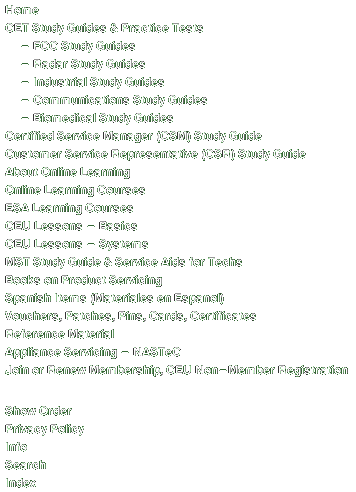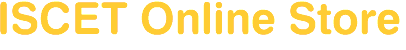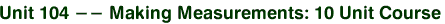Covers units of measurement used in commercial and industrial applications. Examines all aspects of basic measurements, concepts, and procedures, including accuracy and tolerance. Discusses techniques and devices for comparison measurements (dial indicators and gauge blocks). Shows common methods for measuring volume, motion, force, temperature, fluid flow, and electricity. Explains how to use scales and rules, combination calipers, and micrometers. Includes the following individual lessons:Lesson 104.1 -- Units of Measurement; Lesson 104.2 -- Metric Measurement; Lesson 104.3 -- Linear Measurement; Lesson 104.4 -- Comparison/Surface Measurement; Lesson 104.5 -- Measuring Bulk Materials; Lesson 104.6 -- Measuring Motion; Lesson 104.7 -- Measuring Forces; Lesson 104.8 -- Measuring Temperatures; Lesson 104.9 -- Measuring Fluids; Lesson 104.10 -- Measuring Electricity.080\$89.00